# Introduction¶

In the following solution, we will go through the pipeline that achieved the baseline result you saw on the competition platform. Therefore, careful readers will found several things that can be improved and others left apart for further discussions.

# Free Spoken Digit classification¶

In :
import os
import numpy as np
import matplotlib.pyplot as plt


In :
dev_data_path = "./dataset/dev/"
eval_data_path = "./dataset/eval/"


First of all it is better to define a function to load the data from the file system, with the structure specified in the laboratory text.
In this function you can use the wavfile package from scipy.io to read the .wav audio files.

Loading the data exploiting the wavfile.read() function gives you two main information:

1. The sampling rate of the signal (in samples/sec)
2. The array with the amplitudes of the signal recorded for each sample
In :
from scipy.io import wavfile

files = os.listdir(data_path)
wav_files = [file for file in files if file.endswith(".wav")]
X = [None] * len(wav_files)
y = [None] * len(wav_files)
SR = [None] * len(wav_files)

for file in wav_files:
file_name = file.split(".")
if phase == "dev":
f_id, label = tuple(file_name.split("_"))
elif phase == "eval":
f_id, label = file_name, float("NaN")
else:
raise Exception(f"Error - phase '{phase}' not recognised.")

f_id, label = int(f_id), float(label)

sr, data = wavfile.read(os.path.join(data_path,file))  # this return sr: sample rate, data: audio as array

X[f_id] = data.astype(np.float32)
SR[f_id] = sr
y[f_id] = label

return X, y, SR


Then use the function defined above to load the development data into memory

In :
dev_X, dev_y, dev_SR = load_data(dev_data_path, phase="dev")
dev_X[:5], dev_y[:5]

Out:
([array([ 14.,  21.,   0., ...,   3., -48., -80.], dtype=float32),
array([-2., 12.,  1., ...,  3., -3.,  3.], dtype=float32),
array([-9., -7., -5., ..., -5., -1., -7.], dtype=float32),
array([-256., -512.,    0., ...,    0.,    0.,    0.], dtype=float32),
array([-20.,  10.,  26., ...,  -3.,   5., -10.], dtype=float32)],
[4.0, 7.0, 5.0, 2.0, 3.0])

Similarly you can load also the evaluation data into memory.
Remember that the evaluation data does not provide the ground truth labels.

In :
eval_X, _, eval_SR = load_data(eval_data_path, phase="eval")
eval_X[:5]

Out:
[array([   0.,    0., -512., ...,    0., -256., -256.], dtype=float32),
array([  6.,   0.,  -3., ..., -11.,  -2., -20.], dtype=float32),
array([-580.,  153., -668., ..., -360., -339., -343.], dtype=float32),
array([-2., -6.,  0., ..., 23.,  9., 22.], dtype=float32),
array([-1.,  1., -5., ...,  1., -4.,  2.], dtype=float32)]

## Preprocessing¶

Once the data has been loaded, it has to be preprocessed in order to extract the most valuable features that can help us to train the classification model.

When dealing with digital signals two main analysis approaches can be exploited:

• Analysis in time domain
• Analysis in frequency domain

Only the analysis in time domain, that allows you to reach the baseline score on the evaluation set, is proposed in the following solution.

In particular, to perform the preprocessing steps that will transform the input signals into feature vectors, a windowed descriptive statistical analysis can be performed. The signal can be split into chunks and they can be characterized by means of statistical measures (e.g. mean, standard deviation). Doing so you can characterize each signal only with their more relevant information, reducing the dimensionality of the data and simplifying the classifier's training process.

### Data normalization¶

Before applying the preprocessing however, some considerations have to be done:

• Were the audios recorded in the same conditions?
• Do the audios have the same duration?

To answer these questions we can perform some simple checks and adopt visualization techniques to look our data.

In :
SR = dev_SR
print(f"Sampling rate = {SR} [sample/s]")
print("Do the sampling rate is always the same for all the development recordings?",
all([sr == SR  for sr in dev_SR]))
print("Do the sampling rate for the evaluation set is equal to development set?",
all([sr == SR  for sr in eval_SR]))

Sampling rate = 8000 [sample/s]
Do the sampling rate is always the same for all the development recordings? True
Do the sampling rate for the evaluation set is equal to development set? True


With the above check we assure that the sampling rate used to record the audios is equal for all the samples. Thus we can plot the audio waves showing the duration of the audios in seconds.

In :
fig, ax = plt.subplots(1,1,figsize=(12,8))

ax.plot(dev_X) # Plot the first signal

# To show time on the x axes
ax.set_xticks(np.arange(0, len(dev_X), 500))
ax.set_xticklabels([sample/SR for sample in np.arange(0, len(dev_X), 500)])

ax.set_ylabel("Amplitude")
ax.set_xlabel("Time [s]")
ax.set_title("Single audio sample")

plt.show()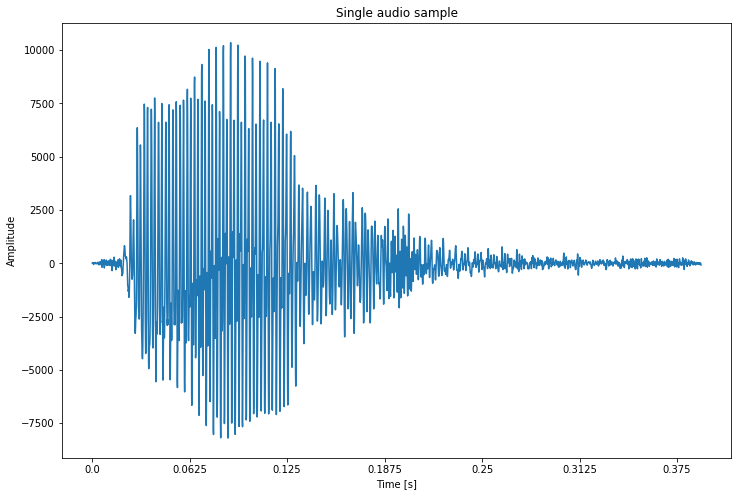In :
def plot_audio_samples(X):
lengths = []
fig, ax = plt.subplots(1,1,figsize=(12,8))

for idx in range(0, len(X), 10):
ax.plot(X[idx], alpha=0.5)
lengths.append(len(dev_X[idx]))

# To show time on the x axes
ax.set_xticks(np.arange(0, max(lengths), 500))
ax.set_xticklabels([sample/SR for sample in np.arange(0, max(lengths), 500)])
return ax

ax = plot_audio_samples(dev_X)
ax.set_ylabel("Amplitude")
ax.set_xlabel("Time [s]")
ax.set_title("Multiple audio samples")
plt.show()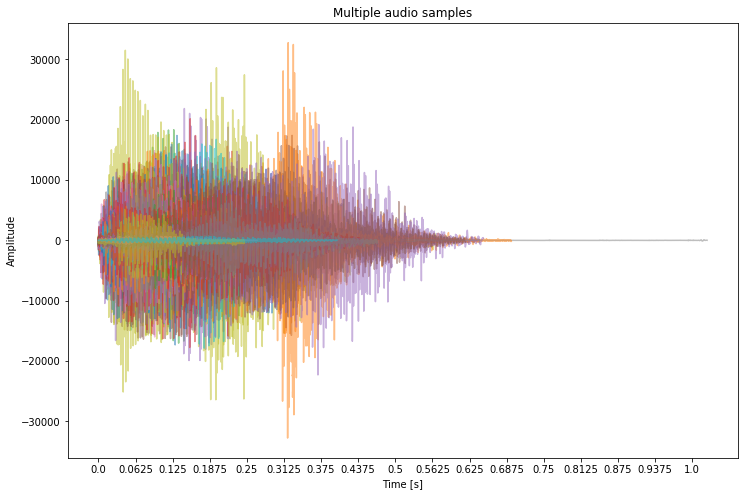### Amplitude normalization¶

From to the visualization of the audio samples it is possible to notice that the vocal samples has very different amplitudes in many cases. This is due to different recording conditions such as the sensibility of the microphone or the recording volume and so on.

To partially overcome these issues it is possible to normalize the amplitude of each recording exploiting the standardization.

In :
from scipy.stats import zscore

def normalize_data(X):
return [zscore(x) for x in X]

dev_X = normalize_data(dev_X)
eval_X = normalize_data(eval_X)

In :
ax = plot_audio_samples(dev_X)
ax.set_ylabel("Normalized amplitude")
ax.set_xlabel("Time [s]")
ax.set_title("Normalized audio samples")
plt.show()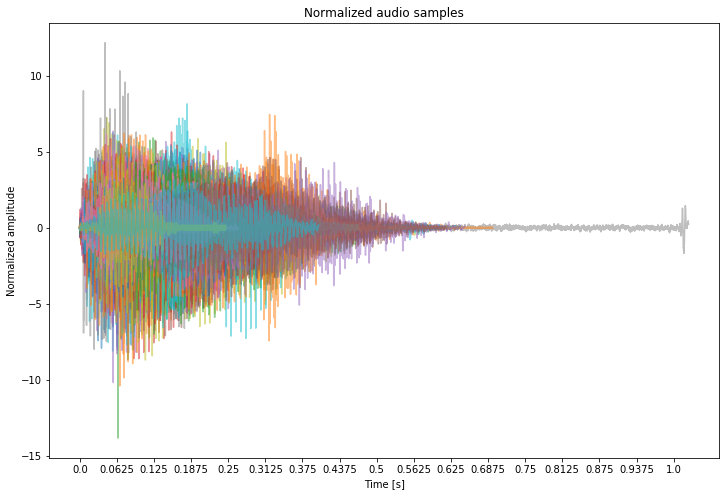### Length normalization¶

Also the duration of the audios is very different. It is possible to inspect the distributions of the lengths in both dev and eval sets to check if any preprocessing is required.

In :
dev_lengths = [len(x) for x in dev_X]
eval_lenghts = [len(x) for x in eval_X]

plt.hist(dev_lengths+eval_lenghts)
plt.title("Audio samples length distribution")
plt.xlabel("Length")
plt.ylabel("# occurrences")
plt.show()
plt.close()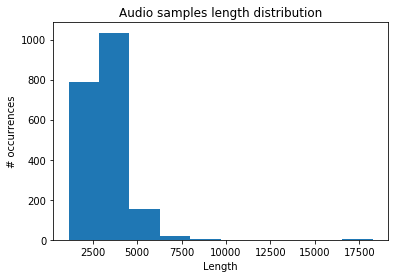From the histogram above it is possible to notice that there is a small number of samples that has a length of around 17500. For example this can be caused by microphones left recording. These can be considered outliers, but, instead of removing them you can try to cut their length reaching the lengths of the other samples. Of course this is a problem only when dealing with analysis in time domain.

To manage this problem you can consider to define an acceptable threshold length so then you can cut the signals longer than the threshold length and add some pad to the audio signal shorter then the threshold.

Consider that the training and the prediction phase of a classifier in many cases (e.g. Rendom Forest) require that the data has always the same number of features. Thus, the dev and eval data should have the same number of features after the feature computation process.

N.B. Frequency domain can have different kind of problems such as the presence of noisy frequencies.

### Cut¶

To choose the threshold we can inspect the percentiles of the lengths vector. In particular we want to find a threshold that includes most of the data and leave out only the outliers. Let's say we want that at least the 95% of our data should have a length equal or lower than the threshold. Which is the threshold value?

In :
perc = 95

length_95_perc = int(np.ceil(np.percentile(dev_lengths+eval_lenghts, perc)))
print(f"The {perc}% of the data has length lower or equal to {length_95_perc}.")

The 95% of the data has length lower or equal to 5000.


Thus, only 5% of data has a length higher than 5000. This is a suitable value that can be used as length threshold.

Apply the cut to both dev and eval data with the threshold value previously defined.

In :
dev_X = [x[:length_95_perc] for x in dev_X]

eval_X = [x[:length_95_perc] for x in eval_X]


Now, looking at the distribution of the signal lengths you can see that they are very well distributed.

In :
dev_lengths = [len(x) for x in dev_X]
eval_lenghts = [len(x) for x in eval_X]

plt.hist(dev_lengths+eval_lenghts)
plt.title("Audio samples length distribution")
plt.xlabel("Length")
plt.ylabel("# occurrences")
plt.show()
plt.close()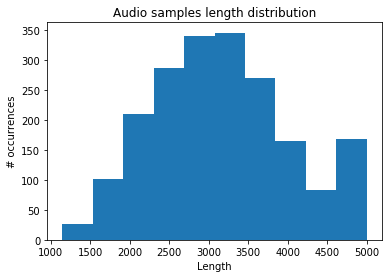Padding all the audio samples with zeros allows every signal to have the same length. In the case of audio sample, including zeros at the end of the recording has a specific meaning: since the values in the array are representing the amplitudes of the signal at each timestamp, the value zero represent the silence.

In :
dev_X = [np.pad(x, (0,length_95_perc-len(x)), constant_values=0.0) for x in dev_X]
eval_X = [np.pad(x, (0,length_95_perc-len(x)), constant_values=0.0) for x in eval_X]


### Window size¶

In order to perform a windowed analysis the size of the window should be carefully analyzed. In this specific case, the size of the window can be derived by the domain.
In fact, studies proved that phonetic elements have specific characteristics. The paper Linguistic uses of segmental duration in English: Acoustic and perceptual evidence highlights that in average unstressed vowels take 70 ms while long consonants such as s can reach a duration of about 200 ms.

In this work we take in consideration a window size that is 1/5 of the maximum duration of 200 ms to consider also short consonant and vowels. For these reasons the window size has been selected to be equal to 40 ms and the number of the corresponding samples has been taken into account accordingly to the sampling rate.

In :
step = int(SR * 0.04) # steps of 40 ms
step

Out:
320

Then, it is possible to visualize the windows with the specified step.

In :
fig, ax = plt.subplots(1,1,figsize=(12,8))
for idx in range(0, len(dev_X), 10):
ax.plot(dev_X[idx], alpha=0.5)

for s in range(0,length_95_perc+step,step):
ax.axvline(s, color="red", linewidth=2)
plt.show()
plt.close()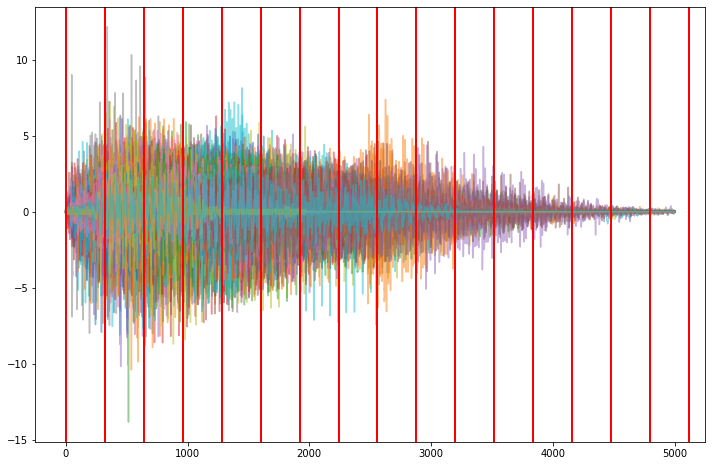### Compute statistical features¶

After normalization phase all the audio samples has the same length. The windows size has been specified to inspect each phonetic element. Thus, it is necessary to define the features that need to be extracted from each window. A small set of possible features that can be calculated is:

• Mean
• Standard deviation
• Min value
• Max value
• Root mean square

Each window will be characterized by the statistics reported above. There are also many other indexes that you can take into account to perform this analysis, the one reported above are the one used to obtain the baseline score.

In the next cell the functions to transform the input signals into statistical features, performing a window analysis with the selected window size, are defined.

In :
def RMS(a):
return np.sqrt(np.mean(np.power(a, 2)))

def compute_signal_statistical_features(x, max_len, step):
feat_x = []
for idx in range(step, max_len+step, step):
seg_x = x[idx-step:idx]
if len(seg_x)>0:
feat = [np.mean(seg_x),
np.std(seg_x),
np.min(seg_x),
np.max(seg_x),
RMS(seg_x)]
else:
feat = [0.0, 0.0, 0.0, 0.0, 0.0]

feat_x.append(
np.array(feat)
)

return np.nan_to_num(np.hstack(feat_x))

def compute_statistical_features(X, max_len, step):
features_X = []
for x in X:
f_x = compute_signal_statistical_features(x, max_len, step=step)
features_X.append(f_x)
return np.vstack(features_X)


Apply the transformation to dev dataset

In :
feat_dev_X = compute_statistical_features(dev_X, length_95_perc, step=step)
feat_dev_X.shape

Out:
(1500, 80)

It is possible to visualize some of the statistical features computed in the feature engineering process. In particular the following plots show the mean and the standard deviations of sample of signals for each class.

In :
fig, ax = plt.subplots(2,5,figsize=(16,12))

for c in np.unique(dev_y):
c_feat_dev_X = feat_dev_X[dev_y == c]
idx = int(c/5)
idy = int(c%5)

for i in range(0,10):
ax[idx, idy].fill_between(x= np.arange(len(c_feat_dev_X[i,0::5])),
y1= c_feat_dev_X[i,0::5]-c_feat_dev_X[i,1::5], # The mean minus the standard deviation
y2=c_feat_dev_X[i,0::5]+c_feat_dev_X[i,1::5], # The mean plus the standard deviation
alpha=0.1)
ax[idx, idy].plot(c_feat_dev_X[i,0::5]) # The mean values
ax[idx, idy].set_title(f"Class {c}")
plt.show()
plt.close()